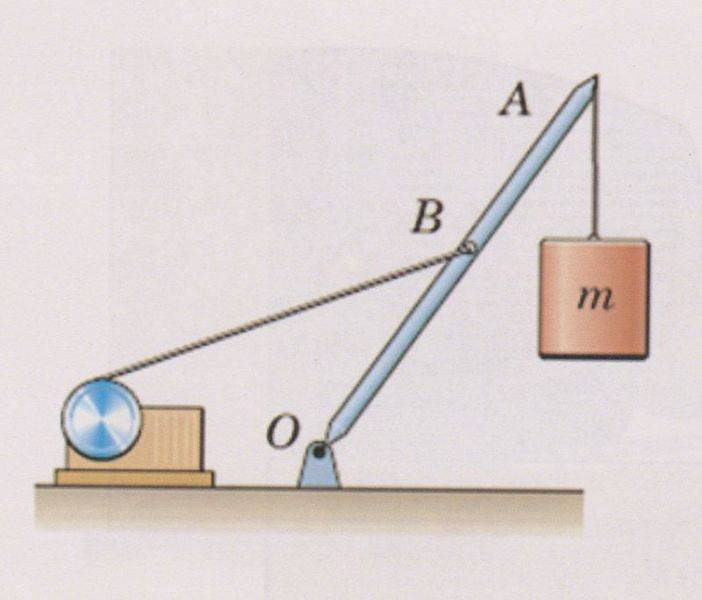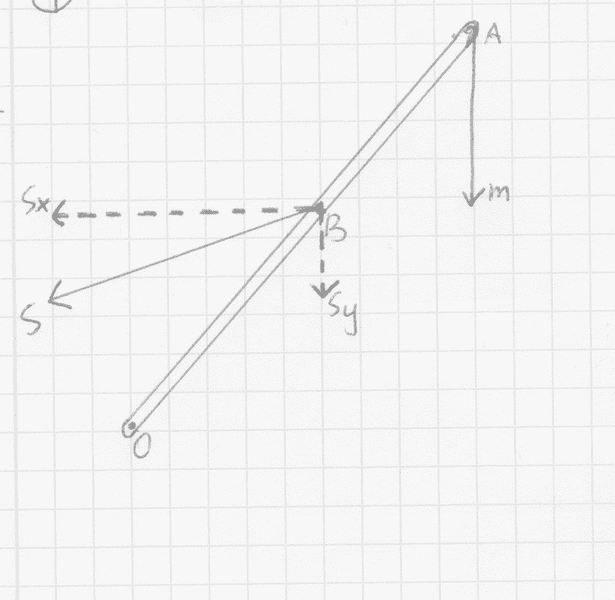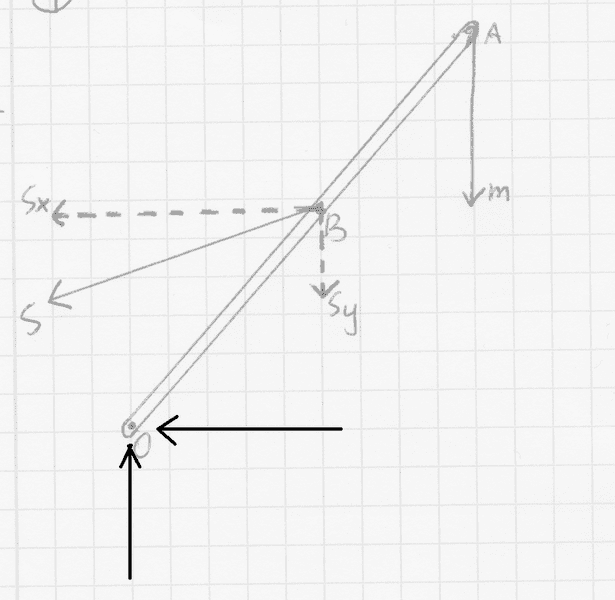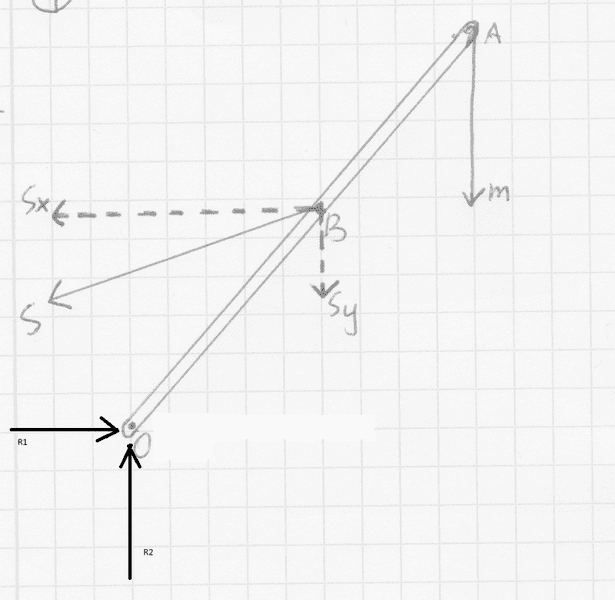# Statics problem involving several forces

There isn't much data, I'm just supposed to simplify the drawing of the body OA and find the forces. The body has a mass m and is connected to the ground with a moving joint in O.Below I post the drawing that I made. I changed the line into a force S, which I then divided into Sx and Sy. I don't know if there is anything else that I can do, but that's how far I went as for now.ΣF=0
ΣM=0

## The Attempt at a Solution

ΣFx=> Sx=0
ΣFy=> Sy+m=0
Mo= Sx-Sy+m=0, I replace Sx with 0 (equation 1) => -Sy+m=0

I really hope someone will help me out on this one, because I'm totally stuck and don't know what else to do. Thank you in advance.

Are any values given?
Did you consider the reaction at the hinge?

•mechanim
Are any values given?
Did you consider the reaction at the hinge?

Thank you for such quick reply!
No, there are no values, the first drawing is all I got. About the hinge - that's where I think the moment (Mo) is, meaning that the rotation happens around the hinge. Does that sound reasonable?
Also, sorry if I use weird names for things sometimes, but I don't study in English, so I'm translating most things by myself.

Yes the rotation does happen around the hinge. But there will also be vertical and horizontal reactions at the hinge point

•mechanim
Thank you!
In this case, the drawing would look something like that:And if the vertical force is F1 and horizontal force is F2, then the calculations would change to:

ΣFx=> -Sx - F2 = 0
ΣFy=> F1 - Sy - m = 0
Mo= m+Sy-F1+F2+Sx=0

Am I right?
One more problem that I noticed with such exercises is that I get confused with signs, I know that if a force is heading left or downwards, it's negative and that a moment that is counterclockwise is also negative, but I still get confused when it comes to calculations. Are the calculations above correct (more or less)?

I just thought of something, here's the latest drawing I just made:I also decided to call the distance between A and O "a" and the distance between B and O "b".

In this case:
ΣFx: R1-Sx=0 => R1=Sx
∑Fy: R2-Sy+m=0 (I'm not sure if it should be + or - before m though)
Mo: m*a+R1+Sy*b-Sx*b+R2=0 (I replace R1 with Sx, since that was the result of the first equation)
ma+Sx+Sy*b-Sx*b+R2=0​

And I'm stuck again, but I hope I moved the exercise forward a bit.

BvU
Homework Helper
You are making progress.
A few details:
• The exercise clearly states the body has mass m. That means the downward force there is not m (m is expressed in kg, force in N).
• Torque is a pseudovector, ##\vec \tau = \vec r \times \vec F## . If you have a right-handed cartesian coordinate system with x to the right, y up then counterclockwise corresponds to positive in the z-direction. Better get that straight, work it out, draw it, visualize it and internalize it, or else it is going to haunt you forever in physics, engineering, math and what have you.
• Post #6 is a lot more sensible than #5.
As to the exercise:
• simplifying means you can draw OA as a simple line too.
• finding the forces is a bit obscure as a description of what is wanted. There's three of them and you found them. Even worked out components in horizontal and vertical directions. But if I were the composer of this exercise, I'd want quantitative symbolic expressions for equilibrium (your thread title says statics, the exercise doesn't mention equilibrium !?)
To come to useable expressions, you can use OA and OB for lengths (or give them a name). There's two more degrees of freedom that haven't gotten a name yet, but are useful in working out your equations when decomposing the forces.

In this case ΣFx: R1-Sx=0 => R1=Sx ##\qquad## OK for magnitude, but it's better to write R1 + Sx = 0 (that's what your relevant equation says) so that the signs come out correctly.

∑Fy: R2-Sy+m=0 (I'm not sure if it should be + or - before m though) ##\qquad## see first comment, and it's strange you have -Sy and +m

Mo:
m*a+R1+Sy*b-Sx*b+R2=0 (I replace R1 with Sx, since that was the result of the first equation) ##\qquad## I can claim I don't know what Mo is. A number, a vector ?
Furthermore, you can't add things with different dimensions. This doesn't look good. What does this represent ?

•mechanim

You are making progress.
A few details:
• The exercise clearly states the body has mass m. That means the downward force there is not m (m is expressed in kg, force in N).

I based the drawing on what teacher have shown to us during a lecture, that's exactly how he draws it and puts mass into a drawing. How should I put mass into a drawing?

• finding the forces is a bit obscure as a description of what is wanted. There's three of them and you found them. Even worked out components in horizontal and vertical directions. But if I were the composer of this exercise, I'd want quantitative symbolic expressions for equilibrium (your thread title says statics, the exercise doesn't mention equilibrium !?)

I agree, the whole exercise is 1 line of text and a drawing. The text is simply "simplify the drawing and find the forces". It is also much different from whatever we did during lectures, since there we usually had an angle or something that would help us with the exercise. Here - nothing.

To come to useable expressions, you can use OA and OB for lengths (or give them a name). There's two more degrees of freedom that haven't gotten a name yet, but are useful in working out your equations when decomposing the forces.

In this case ΣFx: R1-Sx=0 => R1=Sx ##\qquad## OK for magnitude, but it's better to write R1 + Sx = 0 (that's what your relevant equation says) so that the signs come out correctly.

∑Fy: R2-Sy+m=0 (I'm not sure if it should be + or - before m though) ##\qquad## see first comment, and it's strange you have -Sy and +m

The reason why I wrote -Sy and +m is mostly because I was taught that whatever points to the left or downwards is negative, while everything pointing to the right and upwards in positive.

Mo:
m*a+R1+Sy*b-Sx*b+R2=0 (I replace R1 with Sx, since that was the result of the first equation) ##\qquad## I can claim I don't know what Mo is. A number, a vector ?
Furthermore, you can't add things with different dimensions. This doesn't look good. What does this represent ?

Mo is the moment around the point O, again, that's how I've been taught to write it down.

So, if I understand correctly, the moment would be:
Mo=Rx+Sx*OB-Ry-Sx*OB (according to the teacher all forces that want the body to revolve counterclockwise have to be negative, that's why I write "-" before all such forces).

Last edited:
BvU
Homework Helper
Mass is a dimension. We express it in kg, our unit of mass.
Force is another dimension. We express it in N, our unit of force. 1 N = 1 kgm/s^2. Its dimension expressed in more fundamental dimensions is mass * length / time^2 .

So if you draw a situation, you mark a mass m. But if you draw a force diagram, the marking should be mg. Now life becomes difficult: a force is a vector. mg is a vector, conventionally pointing downwards. A y-axis is conventionally pointing upwards (or a z axis in other problems) so mg should have a negative value in a force balance. m is positive definite, so either write mg next to something that is clearly pointing downwards , or (better) write -mg, or (even better) write ##m\vec g## for the force and -mg if you want the vertical component. This is because we always say g = 9.8 m/s2 . We know things don't fall upwards.

The angles were what I was fishing for in the degrees of freedom sentence. Give them names and you can work out the thing quantitatively.

The reason why I wrote -Sy and +m is mostly because I was taught that whatever points to the left or downwards is negative, while everything pointing to the right and upwards in positive.
Ah! (teaser:) so m is pointing upwards ? ;) I much prefer ∑Fy: R2+Sy+mg=0 but you already know that.

an aside here: that means that the angle you use for the cable is in the range ##[\pi,{3\over2}\pi]: both sine and cosine have a minus sign.
Now for the torque around O. You Still have Rx and Ry as terms in there But they are forces, not torques. And m (mg) is missing...
Aren't you missing something about these torques ? An angle, perhaps ?

•mechanim
Mass is a dimension. We express it in kg, our unit of mass.
Force is another dimension. We express it in N, our unit of force. 1 N = 1 kgm/s^2. Its dimension expressed in more fundamental dimensions is mass * length / time^2 .

So if you draw a situation, you mark a mass m. But if you draw a force diagram, the marking should be mg. Now life becomes difficult: a force is a vector. mg is a vector, conventionally pointing downwards. A y-axis is conventionally pointing upwards (or a z axis in other problems) so mg should have a negative value in a force balance. m is positive definite, so either write mg next to something that is clearly pointing downwards , or (better) write -mg, or (even better) write ##m\vec g## for the force and -mg if you want the vertical component. This is because we always say g = 9.8 m/s2 . We know things don't fall upwards.

The angles were what I was fishing for in the degrees of freedom sentence. Give them names and you can work out the thing quantitatively.

Ah! (teaser:) so m is pointing upwards ? ;) I much prefer ∑Fy: R2+Sy+mg=0 but you already know that.

an aside here: that means that the angle you use for the cable is in the range ##[\pi,{3\over2}\pi]: both sine and cosine have a minus sign.​

Now for the torque around O. You Still have Rx and Ry as terms in there But they are forces, not torques. And m (mg) is missing...
Aren't you missing something about these torques ? An angle, perhaps ?

About the mass: that just shows how confused I am I guess... ;)
I know there should be an angle there, but it is not given. That's what confused me the most in the beginning, since most cases I have seen so far had at least one angle given. So, can I use sine and cosine in the exercise even though the angles are not given?
I'm also still not sure if I understand why the equation should be ∑Fy: R2+Sy+mg, while R2 i pointing upwards and the other two are pointing downwards. In this case, shouldn't it be ∑Fy: R2-Sy-mg?

The equations could look like that then:
∑Fy: R2-Sy-mg=0, so: R2=Sy+m
∑Fx: R1-Sx=0, so: R1=Sx
Mo: R1 + Sy*OB + mg*OA - R2 - Sx*OB=0
so if I replace R1 and R2 with the results from equations Fy and Fx:
Sx+Sy*OB+mg*OA-(Sy+m)*OB=0
Is that correct?

Last edited:
BvU
Homework Helper
What is the torque around O as a consequence of R1 (or R2, for that matter) ? In other words: how can R1 cause the beam to rotate around O ?

I'm also still not sure if I understand why the equation should be ∑Fy: R2+Sy+mg, while R2 i pointing upwards and the other two are pointing downwards. In this case, shouldn't it be ∑Fy: R2-Sy-mg?
It should be a sum. ∑Fy = 0 is what the equation for static equilibrium says. A sum is one plus the other plus ... etcetera. That some of these forces point in the other direction means the value of that term in the summation is negative.

Example : ##+m\vec g_y = +m * -|\vec g| = - m * 9.8 ## kg m/s2.
Other example: ##+S_y = + |\vec S| \sin\theta ## , a sine which, as we saw (right?), is negative. (because ##\theta=0## means pointing horizontally in the +x direction, as all physicists agree with all mathematicians and all students ;) )

And the beam makes an angle ##\phi## with the +x direction. That's the other angle I was fishing for. Now: what can we use that one for ? (think torque).

In short: no, we are not where we want to be, yet.

•mechanim
Thank you for the reply, BvU! However, I have to say that I read it and I'm quite lost. I know this may sound like a stupid question, but do I simply replace each "-" with a "+" in all three equations? Does it mean that no matter where the vector is heading, I just put "+" in the equation and then just decide if the result should be negative or positive?
Also, I'm still a bit confused with the angles - should I use them in this exercise? They are not given, so how can I do it?

What is the torque around O as a consequence of R1 (or R2, for that matter) ? In other words: how can R1 cause the beam to rotate around O ?

R1 wants to rotate the body clockwise, while R2 wants to turn it counterclockwise. There is no distance between the forces and the point O, because the forces run through the point. Am I correct?

BvU
Homework Helper
You don't "just decide". A force component has a direction that determines the sign. ##\vec g## is down, so if y+ is up, the y component of that force is ##\ ##- m * |g|
It DOES matter where the vector is pointing.

You can use the angles by describing them and giving them a name. I've already unambiguously described a ##\theta## and a ##\phi##.

In post #7 I tried to bring across that a force is not a torque. For a torque you need a force and an "arm" : You cannot open a door by pressing against the hinge, however hard you push. R1 and R2 can not change the beam angle ##\phi## wrt x+.

The torque from mg around O is not equal to OA times mg with a negative value (negative, because it wants to turn the beam clockwise).

Likewise, the torque of Sx is not equal to OB * Sx with a positive value (positive, because it wants to turn the beam in a mathematically positive (counter-clockwise) direction).

•